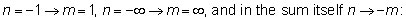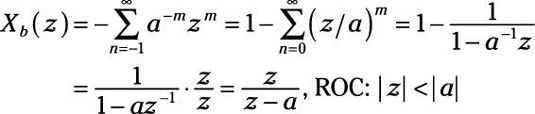##### Signals and Systems For Dummies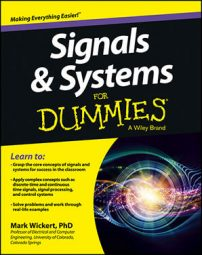The ZT doesn’t converge for all sequences. When it does converge, it’s only over a region of the z-plane. The values in the z-plane for which the ZT converges are known as the region of convergence (ROC).

Convergence of the ZT requires that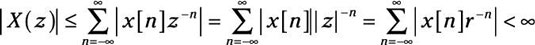The right side of this equation shows that x[n]r-n is absolutely summable (the sum of all terms |x[n]rn| is less than infinity). This condition is consistent with the absolute summability condition for the DTFT to converge to a continuous function of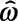Convergence depends only on |z| = r, so if the series converges for z = z1, then the ROC also contains the circle |z| = |z1|. In this case, the general ROC is an annular region in the z-plane, as shown. If the ROC contains the unit circle, the DTFT exists because the DTFT is the ZT evaluated on the unit circle.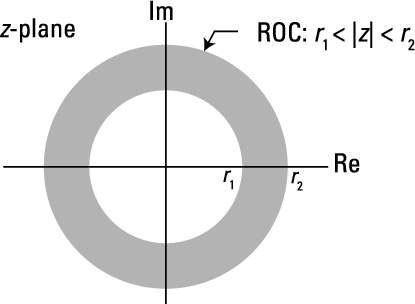The ROC has important implications when you’re working with the ZT, especially the two-sided ZT. When the ZT produces a rational function, for instance, the roots of the denominator polynomial are related to the ROC. And for LTI systems having a rational ZT, the ROC is related to a system’s bounded-input bounded-output (BIBO) stability. The uniqueness of the ZT is also ensured by the ROC.

Consider the right-sided sequence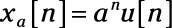The term right-sided means that the sequence is 0 for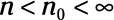and the nonzero values extend from n0 to infinity. The value of n0 may be positive or negative.

To find X(z) and the ROC, follow these steps:

1. Reference the definition to determine the sum: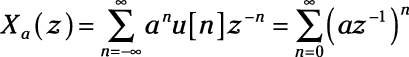2. Find the condition for convergence by summing the infinite geometric series: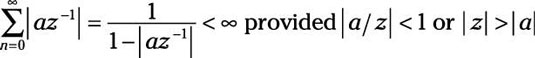Thus, the ROC is |z| > |a|.

3. To find the sum of Step 1 in closed form, use the finite geometric series sum formula: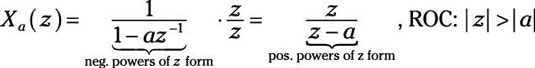The geometric series convergence condition corresponds with the ROC.

Consider the left-sided sequence xb[n] = –anu[–n–1]. The term left-sided means that the sequence is 0 for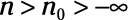To find X(z) and the ROC, write the definition and the infinite geometric series: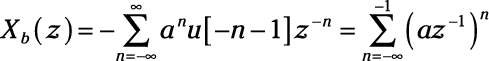If the sum looks unfamiliar in its present form, you can change variables in the sum, an action known as re-indexing the sum, by letting m = –n, then in the limits